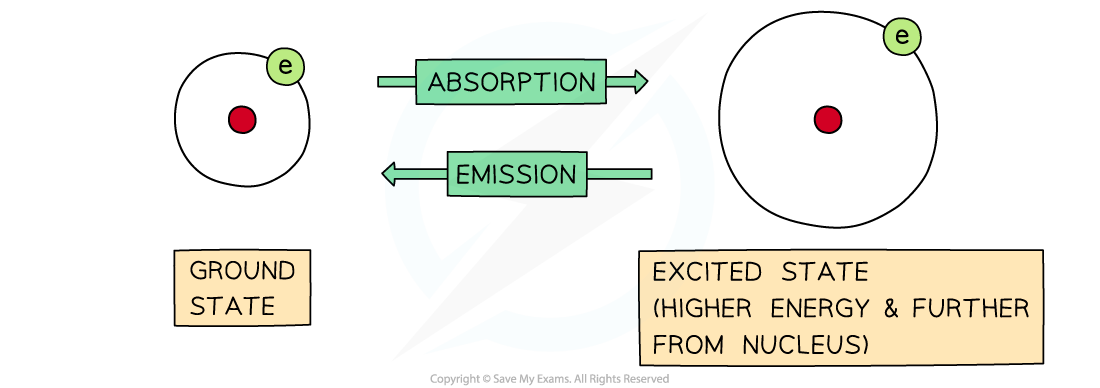# Edexcel A Level Chemistry:复习笔记1.2.2 Quantum Shells

### Developing Electronic Configuration

• Electrons move rapidly around the nucleus in energy shells
• If their energy is increased, then they can jump to a higher energy level
• The process is reversible, so electrons can return to their original energy levels
• When this happens, they emit energy

• The frequency of energy is exactly the same, it is just being emitted rather than absorbed:The difference between absorption and emission depends on whether electrons are jumping from lower to higher energy levels or the other way around

• The energy they emit is a mixture of different frequencies
• This is thought to correspond to the many possibilities of electron jumps between energy shells
• If the emitted energy is in the visible region, it can be analysed by passing it through a diffraction grating
• The result is a line emission spectrum

#### Line emission spectraThe line emission (visible) spectrum of hydrogen

• Each line is a specific energy value
• This suggests that electrons can only possess a limited choice of allowed energies

• These packets of energy are called 'quanta' (plural quantum)
• What you should notice about this spectrum is that the lines get closer together towards the blue end of the spectrum
• This is called convergence and the set of lines is converging towards the higher energy end, so the electron is reaching a maximum amount of energy
• This maximum corresponds to the ionisation energy of the electron
• These lines were first observed by the Swiss school teacher Johannes Balmer, and they are named after him
• We now know that these lines correspond to the electron jumping from higher levels down to the second or n = 2 energy level

#### Successive ionisation energies

• The first electron removed has a low IE1 as it is easily removed from the atom due to the spin-pair repulsion of the electrons in the 4s orbital
• The second electron is more difficult to remove than the first electron as there is no spin-pair repulsion
• The third electron is much more difficult to remove than the second one corresponding to the fact that the third electron is in a principal quantum shell which is closer to the nucleus (3p)
• Removal of the fourth electron is more difficult as the orbital is no longer full, and there is less spin-pair repulsion
• The graph shows there is a large increase in successive ionisation energy as the electrons are being removed from an increasingly positive ion
• The big jumps on the graph show the change of shell and the small jumps are the change of subshellSuccessive ionisation energies for the element calcium

### Filling Quantum Shells

#### Shells

• The arrangement of electrons in an atom is called the electron configuration
• Electrons are arranged around the nucleus in principal energy levels or principal quantum shells
• Principal quantum numbers (n) are used to number the energy levels or quantum shells
• The lower the principal quantum number, the closer the shell is to the nucleus
• So, the first shell which is the closest to the nucleus is n = 1

• The higher the principal quantum number, the greater the energy of the shell and the further away from the nucleus

• Each principal quantum number has a fixed number of electrons it can hold
• n = 1 : up to 2 electrons
• n = 2 : up to 8 electrons
• n = 3 : up to 18 electrons
• n = 4 : up to 32 electrons